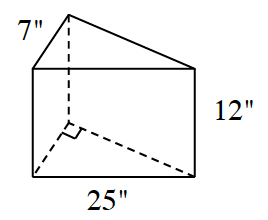Home > CCG > Chapter 11 > Lesson 11.1.4 > Problem11-58

11-58.

Find the volume and total surface area of each solid below. Show all work.

1.Use the Pythagorean Theorem to find the unknown side of the triangle.

Find the area of the top.

Multiply the area of the top by the height to find the volume.

Find the area of each of the sides and sum them.

$\text{Surface Area }= (2)(84) + (12)(7 + 24 + 25) = 840\text{ units}^2$
$\text{Volume = (area base)(height)} = (84)(12) = 1008\text{ units}^3$

1.The volume of a cone is $\frac{1}{3} \pi r^2h$

$\text{Volume}=\frac{1}{3}\pi(5)^2(12)$

$\text{Volume}=100\pi\ \text{un}^3$

Find the area of the base.
Then find the lateral surface areas and add them together.

$\text{base area}=\pi r^2=25\pi$
$\text{lateral surface area }= (π)(5)(13) = 65π$

$\text{Surface area} = 25π + 65π = 90π\text{ units}^2$